CREST IQ Challenge Class 10 Sample Papers

REGISTER NOW

Syllabus:

The IQ Challenge will evaluate students on the following skills:

• Analytical reasoning
• Calculation skills
• Creative thinking
• Information processing
• Lateral thinking
• Observation skills

The questions will be a mix of Basic, Intermediate and Advanced Level.

 Q.1 In a code language, if “ROMANCE” is written as “30”, then how will “GARDEN” be written in that language?
 Q.2 How many parallelograms are there in the figure below?Q.3 From amongst the figures (a), (b), (c), and (d), select the figure which satisfies the same conditions of placement of the dots as in question figure.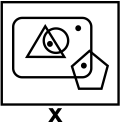Q.4 Which number is on the face opposite 6?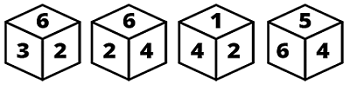Q.5 If FLAT is coded 9645, how will DUMB be coded in the same language?
 Q.6 Find the missing number.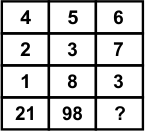Q.7 Directions: In the following question, some statements are given followed by some conclusions. Taking the given statements to be true even if they seem to be at variance from commonly known facts, read all the conclusions and then decide which of the given conclusion logically follows the given statements.Statements:I. Some rats are turtles.II. Some turtles are snakes.Conclusions:I. All snakes are rats.II. Some snakes are not turtles.
 Q.8 If the given figure is folded then which of the following cube is formed?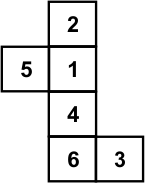Q.9 Read the following diagram carefully and answer the question:Who among the following is only a graduate?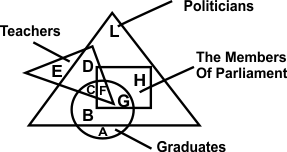Q.10 Find the missing figure in the figure matrix.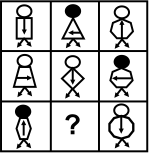Sample PDF of CREST IQ Challenge for Class 10: